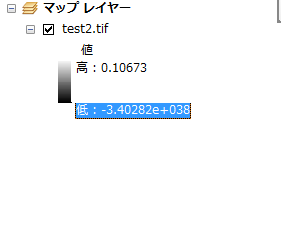# NoData setting differs according to file format in raster save method

1655
7
07-06-2017 12:53 AMNew Contributor II

Hi,

I tried to make masked raster.

At first, making mask raster, and then SetNull for input raster.It's very common process.

All processes is well ,but NoData setting differs according to file format in raster save method....

I've checked following two format.

1. ESRI Gird:output raster has no problem, NoData pixels are not displayed in ArcMap.
2. TIF: Not working NoData value. NoData pixels have values(-3.4028235e+038 or 0). So, NoData pixels are displayed in ArcMap.

Unfortunately,  I need to save in TIF format ,so it is just the problem.

I tried arcpy.env.nodata setting. However, Noting had changed.

What should I do?

# -*- coding: utf-8 -*-
# Import arcpy module
import arcpy
import sys
import os

class LisenceError(Exception):
pass

try:
if arcpy.CheckExtension("Spatial") == "Available":
arcpy.CheckOutExtension("Spatial")
else:
# raise a custom extension
raise LisenceError
except LisenceError:

try:
coheMask = arcpy.sa.Con(arcpy.Raster(coheRas) < ccthresh, 0, 1)
except arcpy.ExecuteError:
print(arcpy.GetMessages(2))

def makeSlopeMask(dem, geoid, angle, heightType, zfactor):
try:
arcpy.env.cellSize = "MINOF"

TMP_SLOPE = arcpy.env.scratchWorkspace + '\\tmp_slope'
arcpy.Slope_3d(dem, TMP_SLOPE, 'DEGREE', zfactor)
except arcpy.ExecuteError:
print(arcpy.GetMessages(2))

try:

outRas = arcpy.sa.Con(arcpy.Raster(lsData) > 1, 0, 1)
return outRas
except arcpy.ExecuteError:
print(arcpy.GetMessages(2))

# Set overwrite option
arcpy.env.overwriteOutput = True

# Arguments
inputRas = sys.argv
coheFlg = sys.argv
coheRas = sys.argv
ccthresh = sys.argv
slopeFlg = sys.argv
dem = sys.argv
zfactor = sys.argv
angle = sys.argv
lsFlg = sys.argv
lsData = sys.argv
output = sys.argv

try:

input_Raster = arcpy.Raster(inputRas)

if coheFlg.lower() == 'true':
else:

if slopeFlg.lower() == 'true':
else:

if lsFlg.lower() == 'true':
else:

outSetNull = arcpy.sa.SetNull(conRas, input_Raster, "VALUE = 0")

outSetNull.save(output)

if arcpy.Exists(TMP_SLOPE):
arcpy.Delete_management(TMP_SLOPE)

except arcpy.ExecuteError:
print(arcpy.GetMessages(2))

Tags (3)
1 Solution

Accepted SolutionsbyMVP Legendary Contributor
7 RepliesbyMVP Legendary ContributorNew Contributor II

I set arcpy.env.nodata prior to using SetNull, trying all method, but I couldn't solve the problem...

(I think arcpy.env.nodata = "NONE" is valid in this case, is my understanding correct?)

I guess arcpy.env.nodata could not work in my script, but why?byMVP Legendary Contributor

you indicate that nodata values are displayed... that should now a symbology issue

http://desktop.arcgis.com/en/arcmap/latest/manage-data/raster-and-images/symbolizing-values-of-nodat...

which doesn't have a solution within arcpy except for using a lyr file.

Can you confirm that the nodata values are truly nodata or are they now being treated as values?New Contributor II

Sorry for my poor explanation

I'm afraid it's not a symbology issue, but, nodata values(i.e -3.40282e+038) are treated as valid value.-3.40282e+038 is treated as valid value...

Of course, I set nodata symbol transparent.

nodata setting in this test data are below

input : 0(32bit float)

0 has two meaning.Therefore I assigned -9998 to nodata of input raster.

But,it didn't work...byMVP Legendary Contributor

this is what the no data value should have been set to, the largest negative number

-3.40282e+038New Contributor II

Thanks Dan!

during process,  no data value is set to.(assigned many values)

So, I use arcpy.SetRasterProperties_management() for every output in script.

Everything goes well!byMVP Legendary Contributor

glad it worked out!  you should close this thread out so that people know a solution was found